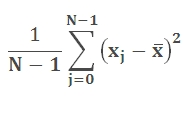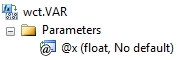# SQL Server sample variance function

VAR

Updated: 28 February 2011

Note: This documentation is for the SQL2008 (and later) version of this XLeratorDB function, it is not compatible with SQL Server 2005.

Use VAR to estimate variance based on a sample, or use VAR to estimate the population variance from a sample of N elements with an unknown mean (the mean being calculated from the sample itself). This function differs from the built-in SQL Server VAR function in that it employs a two-pass and more numerically stable method for calculating the standard deviation and under certain conditions will return a different value than the built-in function.

The equation for VAR is:SyntaxArguments
@x
the values used in the variance calculation. @x is an expression of type float or of a type that can be implicitly converted to float.
Return Types
float
Remarks
·         If you want measure the population variance, then use the VARP function.
·         NULL values are not included in the standard deviation calculation.
·         VAR is an aggregate function and follows the same conventions as all other aggregate functions in SQL Server.
·         If you have previously used the VAR scalar function, the VAR aggregate has a different syntax. The VAR scalar function is no longer available in XLeratorDB/statistics2008, though you can still use the scalar VAR _q.
Examples
SELECT wct.VAR(x) as VAR
FROM (VALUES
(91.3698),
(76.3382),
(74.5692),
(85.2957),
(99.0112),
(86.99),
(70.7837),
(72.834),
(78.1644),
(77.7472),
(66.0627),
(59.781),
(68.4793),
(78.6103),
(59.8621)
) n(x)

This produces the following result
VAR
----------------------
121.668645804095

(1 row(s) affected)

In the following example, we will use demonstrate the difference between the SQL Server VAR calculation and XLeratorDB VAR calculation. Using the XLeratorDB/math SeriesFloat function to create a million rows of randomly generated values between 1,000,000,000 and 1,000,000,010
SELECT ROUND(SeriesValue * 10, 0) + 1000000000 as x
INTO #n
FROM wctMath.wct.SERIESFLOAT(0,1,'',1000000,'R')

SELECT VAR(x) as [SQL SERVER VAR]
,wct.VAR(x) as [XLDB VAR]
,VAR(x-1000000000) as [SQL SERVER VAR adjusted]
FROM #n

DROP TABLE #n

This produces the following result. Your results will vary, since the dataset is randomly generated. The first column shows the built-in SQL Server VAR calculation, the second shows the XLeratorDB calculation and the third column shows the built-in calculation for x minus 1,000,000,000.
SQL SERVER VAR               XLDB VAR SQL SERVER VAR adjusted
---------------------- ---------------------- -----------------------
277965.192653193       8.49671095762951        8.49671095774196

(1 row(s) affected)

### SupportCopyright 2008-2021 Westclintech LLC         Privacy Policy        Terms of Service